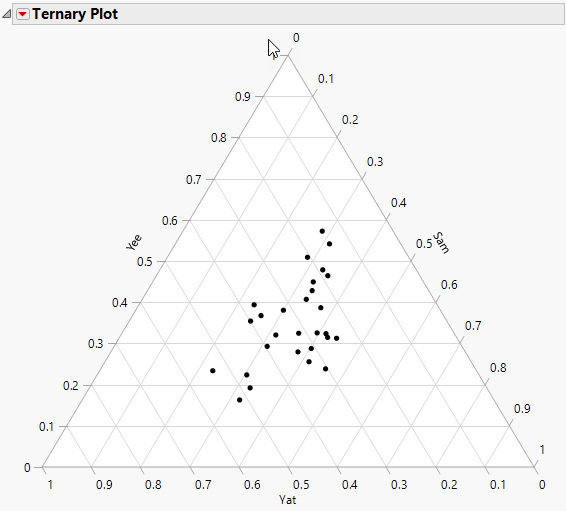Essential Graphing > Ternary Plots > The Ternary Plot
Publication date: 11/29/2021

# The Ternary Plot

Follow the instructions in Example of a Ternary Plot to produce the plot shown in Figure 11.6.

Each of the three sides of a ternary plot represents a proportion of 0%, with the point of the triangle opposite that base representing a proportion of 100%. As a proportion increases in any one sample, the point representing that sample moves from the base to the opposite point of the triangle.

Figure 11.6 The Ternary Plot